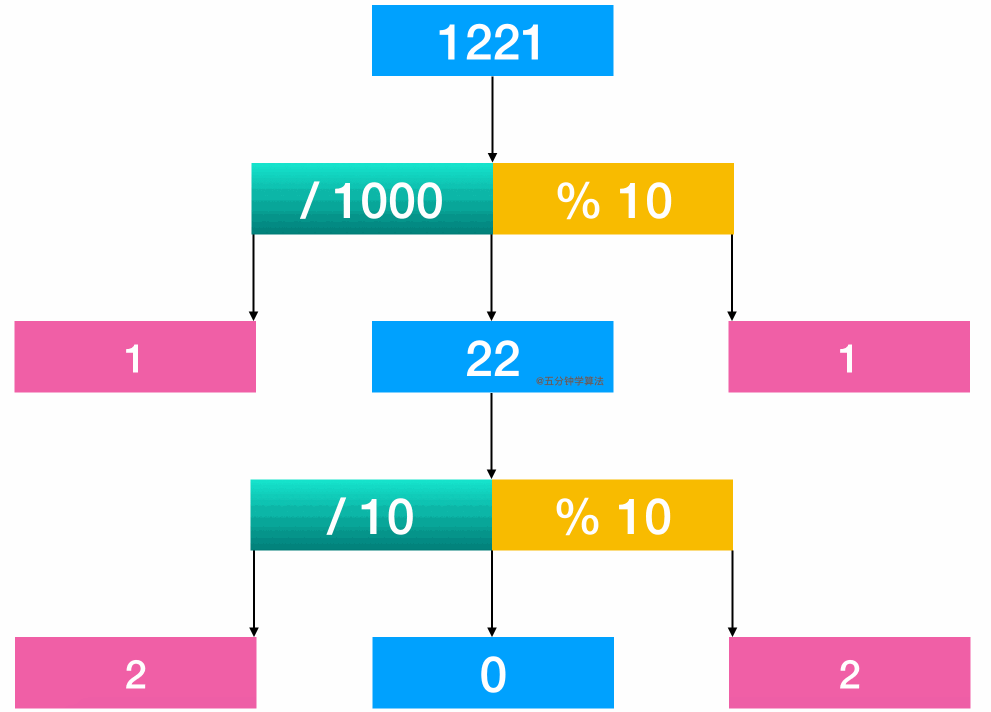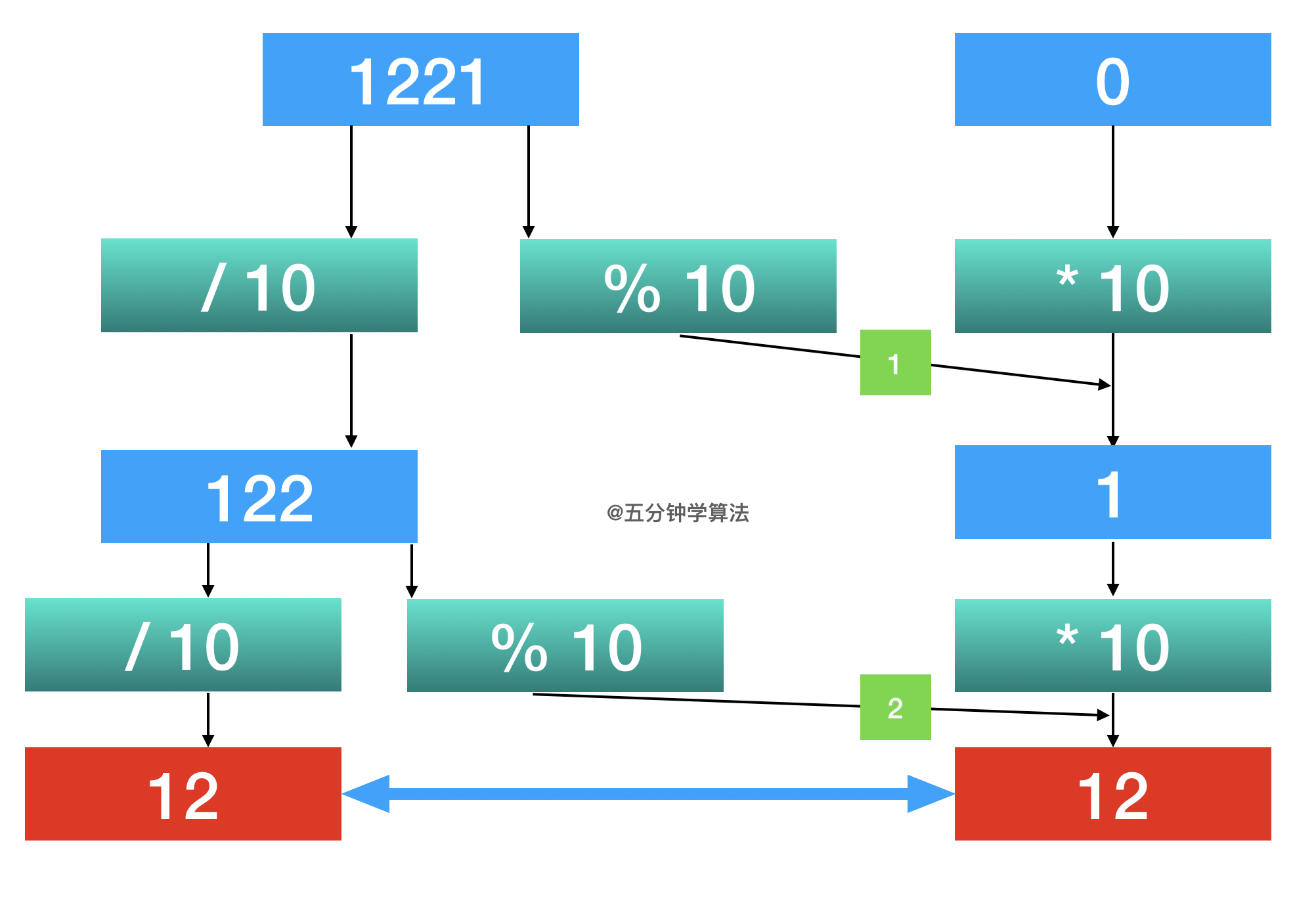## 动画：回文数的三种解法 | 法解种三的数文回：画动

Published on with 859 views and 1 comments

## 题目描述

``````输入: 121

``````

``````输入: -121

``````

``````输入: 10

``````

## 题目解析

### 解法一：普通解法

#### 动画描述#### 代码实现

``````///简单粗暴，看看就行
class Solution {
public boolean isPalindrome(int x) {
String reversedStr = (new StringBuilder(x + "")).reverse().toString();
return (x + "").equals(reversedStr);
}
}
``````

### 解法二：进阶解法—数学解法

• 通过计算 1221 / 1000， 得首位1
• 通过计算 1221 % 10， 可得末位 1
• 进行比较
• 再将 22 取出来继续比较

#### 动画描述#### 代码实现

``````class Solution {
public boolean isPalindrome(int x) {
//边界判断
if (x < 0) return false;
int div = 1;
//
while (x / div >= 10) div *= 10;
while (x > 0) {
int left = x / div;
int right = x % 10;
if (left != right) return false;
x = (x % div) / 10;
div /= 100;
}
return true;
}
}
``````

### 解法三：进阶解法—巧妙解法

• 每次进行取余操作 （ %10），取出最低的数字：`y = x % 10`
• 将最低的数字加到取出数的末尾：`revertNum = revertNum * 10 + y`
• 每取一个最低位数字，x 都要自除以 10
• 判断 `x` 是不是小于 `revertNum` ，当它小于的时候，说明数字已经对半或者过半了
• 最后，判断奇偶数情况：如果是偶数的话，revertNum 和 x 相等；如果是奇数的话，最中间的数字就在revertNum 的最低位上，将它除以 10 以后应该和 x 相等。

#### 动画描述#### 代码实现

``````class Solution {
public boolean isPalindrome(int x) {
//思考：这里大家可以思考一下，为什么末尾为 0 就可以直接返回 false
if (x < 0 || (x % 10 == 0 && x != 0)) return false;
int revertedNumber = 0;
while (x > revertedNumber) {
revertedNumber = revertedNumber * 10 + x % 10;
x /= 10;
}
return x == revertedNumber || x == revertedNumber / 10;
}
}
``````Responses